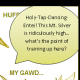# The Unapologetic Mathematician

## Commutator Subgroups

If we have a group$G$, it may or may not be abelian. We can measure how nonabelian it is with the commutator subgroup$\left[G,G\right]$. This is the subgroup generated by all the commutators in$G$. Start with all the elements of$G$ of the form$ghg^{-1}h^{-1}$ with$g$ and$h$ any elements of$G$. If$G$ is abelian, these are all trivial.

If$G$ is not abelian,$\left[G,G\right]$ is a nontrivial normal subgroup of$G$ (verify), so we can form the quotient$G/\left[G,G\right]$. This group is abelian. In fact, there’s a universal property floating around. If we have a group$G$, an abelian group$A$, and a homomorphism$f:G\rightarrow A$, there is a unique homomorphism$s:G/\left[G,G\right]\rightarrow A$ so that$f$ is the composition$G\rightarrow G/\left[G,G\right]\rightarrow^sA$. From this we can see that if$N$ is any normal subgroup of$G$ with$G/N$ abelian, then$\left[G,G\right]\subseteq N$.

We can repeat this construction. Define$G^{(0)}$ to be$G$ itself, and$G^{(n+1)}=\left[G^{(n)},G^{(n)}\right]$. This series of subgroups is extremely important in the classification of groups. If eventually it bottoms out at the trivial subgroup we say the group is “solvable”, which recalls the origins of group theory in finding solutions to polynomial equations. We say a group$P$ where$\left[P,P\right]=P$ is “perfect”. If the series of groups ever hits a perfect group then it stops and will never bottom out, so the original group can’t be solvable.

In 1963, John Thompson and Walter Feit proved that a finite group$G$ with an odd number of elements is solvable. Their paper ran over 250 pages, consuming an entire issue of the Pacific Journal of Mathematics. If you’re up for it, you can read it yourself. This result really got the ball rolling on the project to completely classify all finite simple groups. The classification is considered by many to be complete, spanning tens of thousands of pages in over 500 articles. Since it’s so huge a body, some people think that no one person can check it all over and thus verify the classification. The controversy rages on.

March 14, 2007 -

3.Pingback by Commutator subgroup; quaternions; cyclic groups | Peter's ruminations | September 24, 2011 | Reply